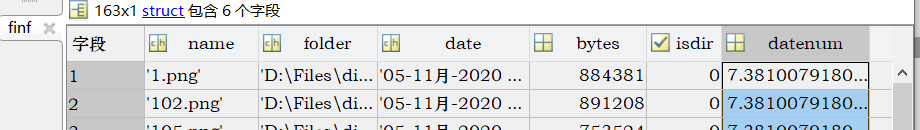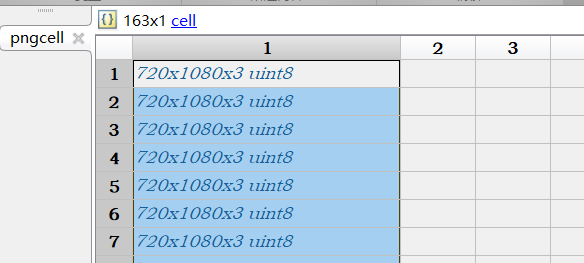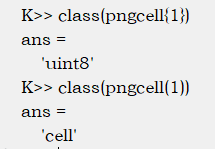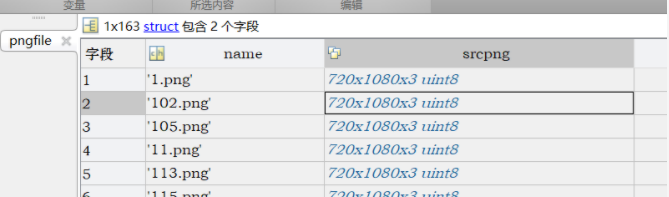• 用于MATLAB图像批处理程序。直接读取指定文件夹图片，并对图片进行等值线操作，处理后的图像可提取出来保存到自己设定的文件夹内。可直接运行。
• 在网上找到的参考代码如下：看的不是很明白，请问大家我怎么样可以把处理后的图像imwrite到其它指定的文件夹了，例如我想保存到这个位置 E:\MATLAB2014\毕业设计\pictures，希望大家可以指导一下，最好给小女子解释...length strcat
• matlab截取图像批处理，批量读取截取图像，使用几张互联网上的身份证图片作为例子截取说明，自动分类保存
• matlab批处理上百张.png图像，目的是将所有图片进行增强（如histeq直方图均衡），然后写入指定文件夹，图像名称不变。 思路： 用dir函数读所有图片的信息并生产一个结构组，dir详见matlab的help 利用name读取每...
在matlab上批处理上百张.png图像，目的是将所有图片进行增强（如histeq直方图均衡），然后写入指定文件夹，图像名称不变。
思路：

用dir函数读所有图片的信息并生产一个结构组，dir详见matlab的help利用name读取每个png文件的图像构成元胞pngcell注意：对cell的操作花括号和圆括号的不同：
通过小括号()里面加下标，访问cell数组中的数据，返回的是对应的cell。
通过大括号{}里面加下标，访问cell数组中的数据，返回的是对应cell的内容。
如对本程序中的pngcell操作：利用dir中的name信息和pngcell的图像信息再构造一个只含文件名和图像数据的结构组pngfile接下来写个循环对图像进行操作就行，可以用imwrite将图像保存至指定位置

代码：
clear all
%读取文件夹中的.png文件
src='D:\Files\diploma_project\dark\darkface163\';
finf = dir([src,'*.png']);
%根据文件夹中的文件格式，选择读取类型，如.jpg、.png等.其中finf是一个结构体数组，...
...包含的darkface下所有png文件的名称、修改时间大小、是否文件夹等属性。

n = length(finf);                   %数组大小,即图片的数量
pngcell = cell(n,1);            %生成n*1的元胞
for k=1:n
filename = [src,finf(k).name]; %构造第k个文件的绝对路径（合并文件路径和文件名）
pngcell{k} = importdata(filename);%将文件夹中的文件每个作为一个元胞按行存储在元胞中
pngfile(k).name= finf(k).name; %创建新结构组，并设定名称
pngfile(k).srcpng=cell2mat(pngcell(k));%将图像数据导入结构组
end
%该节生成了一个含有文件名和图像数据的结构组pngfile，...
...名称排序序是matlab自动读取的字符串排序型，如1,10,111，2，...

%% 直方图均衡批处理
dst='D:\Files\diploma_project\dark\darkface163_histeq\';
for k=1:n
B=histeq(pngfile(k).srcpng);    %直方图均衡
%imshow(B);
imwrite(B,[dst,pngfile(k).name])  %将处理后的图片保存到目标文件夹，命名与源文件相同
end




展开全文• matlab 图像批处理的代码，是我最近学习的结果与总结，希望对大家有用
• imbatchconvert 使用 get_file_list 执行图像批处理。 例子： % 批量裁剪图像 options.Func = 'imcrop'; options.FuncPar = [10 10 40 40]; options.Verbose = true; options.NameSuffix = '_cropped'; src_dir = ...
• matlab开发-正在获取文件列表和图像批处理。类grep和图像批处理功能控制系统
• clc clear all close all for k=2:4 %比如有三张图片 s=strcat('D:\matlab\matlab r2013a\bin\train\',int2str(k-1),'.jpg');%图片路径 I=imread(s); I_gray=rgb2gray(I); %转换为灰度图像 ...
clc
clear all
close all
for k=2:4  %比如有三张图片
s=strcat('D:\matlab\matlab r2013a\bin\train\',int2str(k-1),'.jpg');%图片路径
I=imread(s);
I_gray=rgb2gray(I);                              %转换为灰度图像

s=strcat('D:\matlab\matlab r2013a\bin\train\',int2str(k+3),'.bmp');%保存图片路径
imwrite(I_gray,s);
end
展开全文• 有关图像批处理Matlab程序-pichuli.m 在这个程序中，为要处理的图像编码 然后就可以对这批图像进行处理，具体的处理我没有写，可以根据自己的需要添加
• 有关图像批处理Matlab程序-ctname.m 在这个程序中，为要处理的图像编码 然后就可以对这批图像进行处理，具体的处理我没有写，可以根据自己的需要添加
• 图像的数字化操作 图像的失真校正操作 直方图变换 滤波器设计
• it's a MATLAB question. In other words, the basic MATLAB techniques for batch processing apply to any domain, not just image processing. Contents Step 1: Get a list of filenames ...

Batch processing

From: Steve on Image Processing http://blogs.mathworks.com/steve/?p=61
A couple of months ago I was working with a bunch of pictures I had taken at home. I had about 40 of them, and I needed to crop and resize them all the same way. Naturally, I wrote a MATLAB script to do it.
This experience reminded me that customers sometimes ask "How do I use the Image Processing Toolbox to do batch processing of my images?" Really, though, this isn't a toolbox question; it's a MATLAB question. In other words, the basic MATLAB techniques for batch processing apply to any domain, not just image processing.
Contents

Step 1: Get a list of filenames
Step 2: Determine the processing steps to follow for each file
Step 3: Put everything together in a for loop

Step 1: Get a list of filenames
If you use the dir function with an output argument, you get back a structure array containing the filenames, as well as other information about each file.
Let's say I want to process all files ending in .jpg:
files = dir('*.jpg')
files =
42x1 struct array with fields:
name
date
bytes
isdir

The files struct array has 42 elements, indicating that there are 42 files matching "*.jpg" in the current directory. Let's look at the details for a couple of these files.
files(1)
ans =
name: 'IMG_0175.jpg'
date: '12-Feb-2006 10:49:30'
bytes: 962477
isdir: 0

files(end)
ans =
name: 'IMG_0216.jpg'
date: '12-Feb-2006 11:09:10'
bytes: 1004842
isdir: 0

Step 2: Determine the processing steps to follow for each file
There are four basic steps to follow for each file:
1. Read in the data from the file.
2. Process the data.
3. Construct the output filename.
4. Write out the processed data.
Here's what my read and processing steps looked like:
rgb = imread('IMG_0175.jpg');  % or rgb = imread(files(1).name);
rgb = rgb(1:1800, 520:2000, :);
rgb = imresize(rgb, 0.2, 'bicubic');
You have many options to consider for constructing the output filename. In my case, I wanted to use the same name but in a subfolder:
output_name = ['cropped/' files(1).name] % Use fullfile instead if you want
% multiplatform portability
output_name =
cropped/IMG_0175.jpg

Here's another example of output name construction. You might use something like this if you want to change image formats.
input_name = files(1).name
input_name =
IMG_0175.jpg

[path, name, extension] = fileparts(input_name)
path =
''
name =
IMG_0175
extension =
.jpg

output_name = fullfile(path, [name '.tif'])
output_name =
IMG_0175.tif

Step 3: Put everything together in a for loop
Here's my complete batch processing loop:
files = dir('*.JPG');
for k = 1:numel(files)
rgb = imread(files(k).name);
rgb = rgb(1:1800, 520:2000, :);
rgb = imresize(rgb, 0.2, 'bicubic');
imwrite(rgb, ['cropped/' files(k).name]);
end


展开全文processing filenames output extension
• str = 'C:\'; %输入路径 ...%输入图像格式设置 path = strcat(str, strAll); file = dir(path); %读取文件列表 for ii = 1 : length(file) ImgPath = strcat(str, file(ii).name); Img = imread(Im
str = 'C:\';      %输入路径
strAll = '*.jpg';%输入图像格式设置

path = strcat(str, strAll);
file = dir(path);       %读取文件列表
for ii = 1 : length(file)

ImgPath = strcat(str, file(ii).name);
Img = imread(ImgPath);                 %读入图像
%%%%%%%%%%%%%%%%%%%%%%%
%你的代码
%%%%%%%%%%%%%%%%%%%%%%%%%%
end

展开全文• 图像批处理分析（BASIS）是Matlab框架，用于自动化，存档和运行图像分析，图像处理和机器视觉工作流程。 BASIS是一个完全面向对象的，功能齐全的数据流框架。 简而言之，它使您可以运行存储为.gml文件的DAG。 图形...
• ## 【Matlab】批处理 mat2nii 及 nii2mat

千次阅读 热门讨论 2018-11-28 18:19:51
但是我用的医学图像都是 nii序列 数据处理完需要将 mat 转 nii 格式，matlab 果然比普通程序易懂~~~ 首先需要下载matlab官网的load_nii文件夹（ps：这个程序要放在load_nii文件夹里） 如果只需处理一张（17-21）...
• 有一个图像分块的代码，可以直接将一幅图像分为5*5的小块，代码如下： %[FileName,PathName] = uigetfile('*.*','Select the image'); Im=imread('Bicubic flowers.bmp'); imshow(Im) hold on L = size(Im);...
• 源码很简单如下： clc clear all close all for k=1:62  s=strcat('E:\20151020\',int2str(k-1),'.bmp');  I=imread(s);  I=im2double(I);... %计算灰度图像的阈值  BW=im2bw(I,level);
• matlab 数据实验数据处理，实验生成的txt文档，要求处理成图像，大批量的处理程序
• 虽然一直不想写批处理函数来处理实验，但是批处理的优越性还是在完成编程的那一刻解放了双手，狠狠打了自己一巴掌，真好用。可能我只是懒，或许也不愿动脑子。言归正传，在这篇博文中，编写一个普适性的批处理的框架...
• % Matlab读取envi图像img格式（批处理） (2011-08-11 15:49:05)转载▼ % 标签： 杂谈 % 试验：Matlab读取ENVI标准图像（IMG+Hdr），并计算相关系数。 % 1、 读取ENVI标准图像代码非原创。文件名read_ENVIimagefile....
• 利用matlab 进行批处理抠图，可以选择图像块位置，或者直接进行指定
• 2011-03-04 16:09:31| 分类： 日常所学 | 标签： |字号大中小 订阅 ...例子:处理一文件夹下的所有文件，读取每个文件里的数据并利用相应数据画图，保存每个图片。...%--put all the fil
• 类似于photoshop球面化滤镜，可调整各种参数，可用于图像批处理
• 下面就总结了几种批处理的方法。 在讲解这几种方法之前，先介绍MATLAB中的一个cell，这个cell相当于一个数组，只不过它允许不同的元素是不同的类型的，比如：cell(1,3)  表示预分配1*3的一个矩阵，然后我们就可以...
• MATLAB Image Processing Toolbox 入门教程九】1 implay函数 图像序列是一组与时间或空间位置相关联的图像。...本次所讲解的内容就是MATLAB中如何显示图像序列以及如何对图像序列进行批处理。 1 implay函数 ...计算机视觉
• 今天下载彩色版的书后发现全部都是图片，而且两页都在同一面，浏览的时候太麻烦了，虽然有黑白的pdf（歪歪斜斜），最后用编程解决问题。尊重版权，请买正版书（这本书太老了）。   ...用Matlab即可正则表达式 java
• 利用小波变换 和hsv变换实现图像融合，并且实现批处理
• MATLAB实现mat文件批处理转化为png格式（全网仅有！） 由于部分算法（如边缘检测算法），跑出来的结果图是带有白色边框的，如下图：（跑过程序，做过算法的人都知道） 那么这为后续处理提供了极大的不便，因为空白...人工智能 机器学习 python...

# matlab图像批处理matlab 订阅